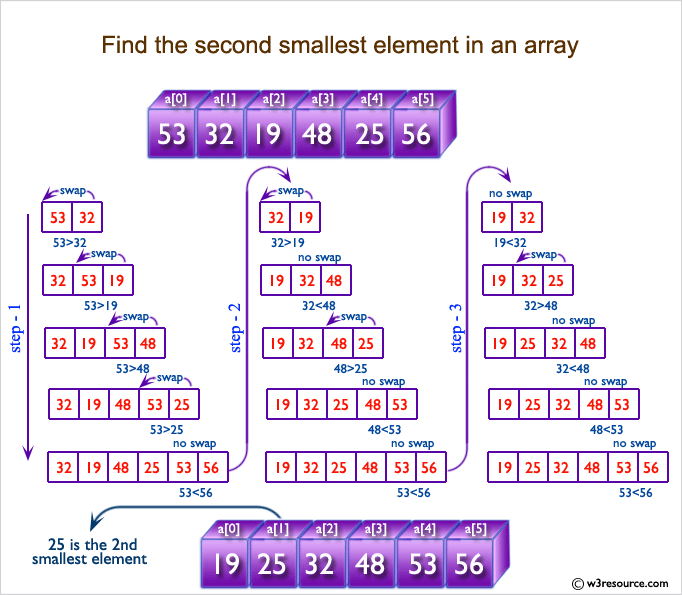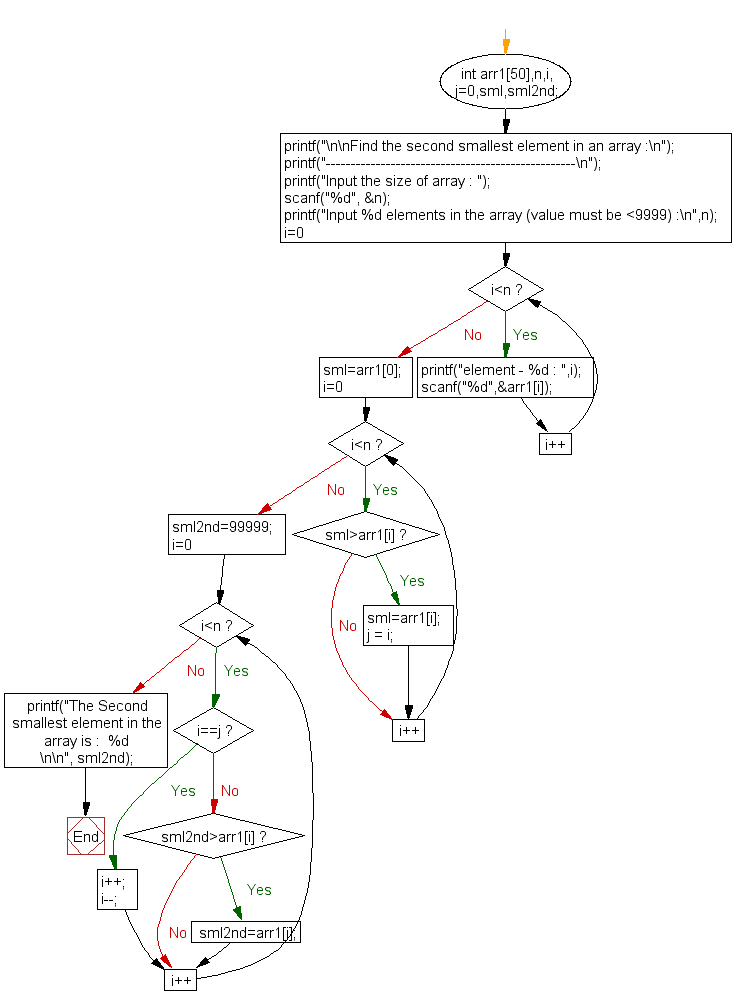﻿ C exercises: Find the second smallest element in an array - w3resource# C Exercises: Find the second smallest element in an array

## C Array: Exercise-17 with Solution

Write a program in C to find the second smallest element in an array.

Pictorial Presentation:Sample Solution:

C Code:

``````#include <stdio.h>

void main()
{
int arr1,n,i,j=0,sml,sml2nd;

printf("\n\nFind the second smallest element in an array :\n");
printf("--------------------------------------------------\n");

printf("Input the size of array : ");
scanf("%d", &n);
/* Stored values into the array*/
printf("Input %d elements in the array (value must be <9999) :\n",n);
for(i=0;i<n;i++)
{
printf("element - %d : ",i);
scanf("%d",&arr1[i]);
}
/* find location of the smallest element in the array */
sml=arr1;
for(i=0;i<n;i++)
{
if(sml>arr1[i])
{
sml=arr1[i];
j = i;
}
}

/* ignore the smallest element and find the 2nd smallest element in the array */
sml2nd=99999;
for(i=0;i<n;i++)
{
if(i==j)
{
i++;  /* ignoring the smallest element */
i--;
}
else
{
if(sml2nd>arr1[i])
{
sml2nd=arr1[i];
}
}
}

printf("The Second smallest element in the array is :  %d \n\n", sml2nd);
}
```
```

Sample Output:

```Find the second smallest element in an array :
--------------------------------------------------
Input the size of array : 5
Input 5 elements in the array (value must be <9999) :
element - 0 : 0
element - 1 : 9
element - 2 : 4
element - 3 : 6
element - 4 : 5
The Second smallest element in the array is :  4
```

Flowchart:C Programming Code Editor:

Improve this sample solution and post your code through Disqus.

What is the difficulty level of this exercise?

﻿